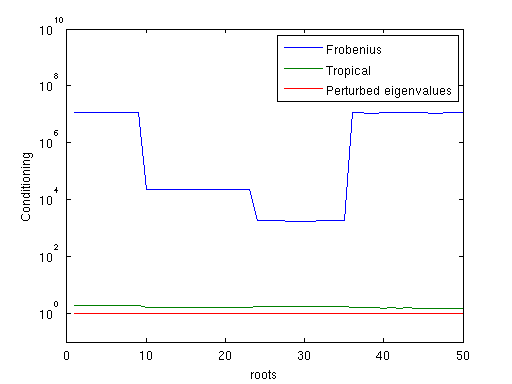# Secular linearization

In this page you will find a brief recap of the numerical experiments carried out in the paper “On a class of matrix pencils equivalent to a given matrix polynomial” and the Matlab code to reproduce them in your environment.

Here you can download the code used to generate all the experiments below: secular-linearization.tar.gz. The examples can be run both in Matlab and in GNU Octave, but for the latter you will need an implementation of condeig.

## Numerical experiments

Here is a brief description of the numerical experiments available in the paper. For a more in-depth description, please refer to the version on arxiv (link missing).

### Random scalar polynomialsA first test has been carried out by measuring the conditioning of the secular companions for a scalar polynomial $p(x) = \sum_{i = 0}^n p_i x^i$ where the coefficients $p_i$ have unbalanced norms. In this case the classical Frobenius companion may be bad conditioned (with respect to the eigenvalue problem) but we show that the secular linearization built using the tropical roots has good conditioning properties. We also show that building a secular companion using as nodes $b_i = \xi_i + \epsilon_i$ with $\xi_i$ the roots of $p(x)$ and $\epsilon_i$ “small” leads to an almost perfectly conditioned problem.

### Random matrix polynomials

As a second experiment, we propose a matrix polynomial similar to the previous scalar case. We have built some random matrix polynomials $P(x) = \sum_{i = 0}^n P_i x^i$ with $P_i \in \mathbb{C}^{m \times m}$ with unbalanced norms. We have then built the Frobenius companion and the secular linearization using as nodes the block-mean of the eigenvalue moduli and the tropical roots. The results are shown in the following graphs.

### NLEVP problems

Some problems from the NLEVP collection have been analyzed. Here are the experimental results for the orr_sommerfeld and the planar_waveguide problem.

### Numerical solution of a PEPAs a final example we have tried to solve a PEP using polyeig and the secular linearization coupled with eig. In the case that we have analyzed, that is defined by integer coefficients, we have solved the PEP with a higher precision and then we have compared the results. It turns out that using the tropical roots to build the secular linearization leads to accurate results with respect to the use of polyeig. You can test this example by using the function Experiment3 in the tarball.